1. Trapezoidal thread size Calculation: (According to the basic size calculation publicity table)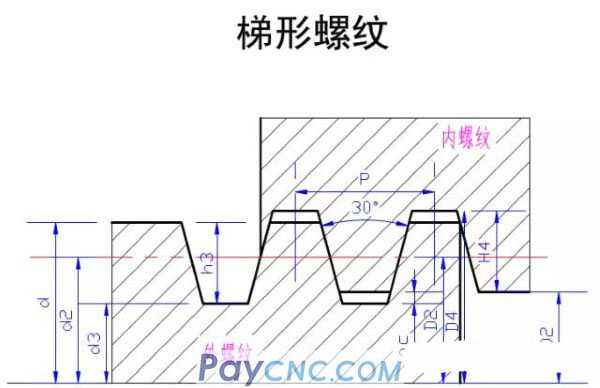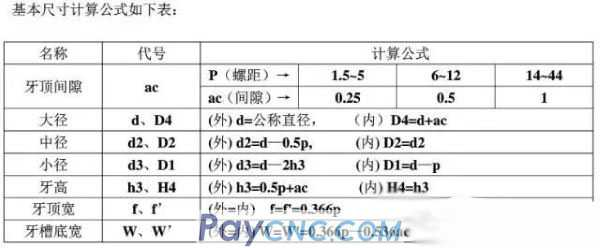Tooth height on one side: h3=0.5p+ac=0.5×6+0.5=3.5mm The trapezoid thread is placed to the minor diameter: d3=d—2h3=36—2×3.5=29mm Tooth crest width: f=f'=0.366×P=0.366×6=2.196≈2.2 Alveolar base width (sharpening width): W=W'=0.366×P—0.536×ac=1.928≈1.93mm Therefore, the width of the sword is set to 2mm, (a few threads larger than 1.93 because of wear)     If the material is hard, such as steel parts, two knives are usually used; 1. ①The first tool is a rough turning tool, (knife sharpening roughly) normal tool setting, the key is to leave a margin for the fine turning tool to repair ②The second tool is a fine turning tool, (the tool must be ground accurately, 0.1 is too large, because it is worn, and it can only be adjusted to size, it has a "ac" gap range margin), normal tool setting (position Z0) ), the key is to set the "Z" direction offset (-0.15~-0.2mm) in the wear bar at the same time, so that both sides of the knife can be polished during finishing.   2. ①For those with higher requirements, use G92 to follow to the size ②Higher attention is required. One is to divide the rough and fine turning, and pay attention to the trimming allowance. For example, leave a margin of 0.1 for the outside of the car and the inside of the boring. After the trapezoidal thread is placed to the size, (because of burrs) Use a turning tool or a boring tool to move it to the size, and finally use a trapezoidal thread cutter to trim it again, so that after the trapezoidal thread is placed, it will feel better and look better. As shown below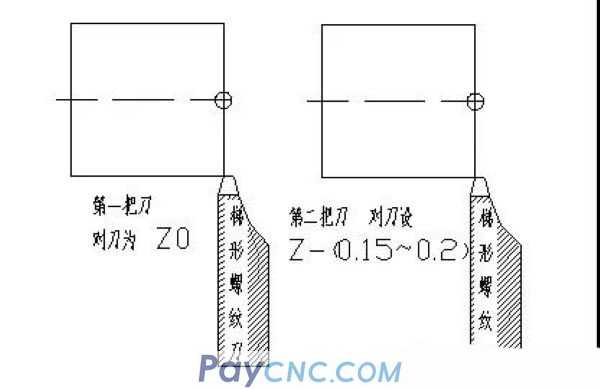Machining Large-pitch Trapezoidal Threads in Layers by Macro Program   1. Parameter table: The variables and meanings used in the macro program [2, 3] are shown in Table 1. Table 1 Variables and their meanings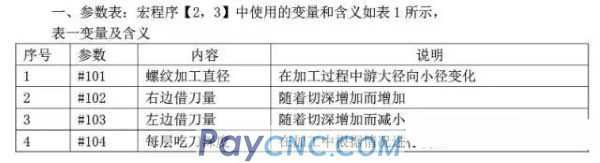Figure 1 External trapezoidal thread  Trapezoidal thread fitting2 Trapezoidal thread turning process analysis     There are many types of machining trapezoidal threads: straight approach, oblique approach, left-right cutting, turning straight grooves, layered methods, and so on. Compared with triangular threads, trapezoidal threads have larger pitch and tooth profile, high precision, and smaller surface roughness values ​​on both sides of the tooth profile, which results in deep cutting, faster cutting and large cutting margins when turning trapezoidal threads. Great cutting resistance. Using the macro program for layered cutting can solve the problems well.     The "layered method" turning trapezoidal thread is actually a comprehensive application of the straight forward method and the left and right cutting method. When turning a trapezoidal thread with a large pitch, the "layered method" usually does not cut the trapezoidal groove at one time, but divides the groove into several layers, and the depth of each layer is determined according to the actual situation. Transform into several shallow trapezoidal grooves for cutting, which can reduce the difficulty of turning. The cutting of each layer adopts the method of turning left and right alternately. The amount of the back tool is very small. The tool only needs to cut along the left and right profile lines. The trapezoidal thread cutter always has only one side edge to participate in the cutting (Figure 2), so that the chip removal is compared. Smoothly, the force and heating of the tool tip have been improved, so higher quality trapezoidal threads can be machined, which is easy to master, short and easy to operate. Figure 2 Layered cutting method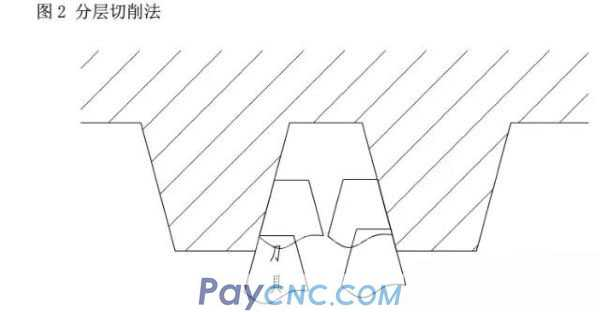2. Size calculation (omitted) 3. Procedure: Taking FanucOi mate TC system as an example, the machining procedure of trapezoidal thread shown in Figure 1 is as follows: O0001; (notching etc. omitted) T0101 M03 S300; Change trapezoidal thread cutter, spindle speed 300r∕min G00 X38 Z5; quickly walk to the starting point M08; cooling on #101=36;Nominal diameter of thread #102=0; Initial value of borrowing tool amount on the right #103=—1.876; the initial value of the borrowing amount on the left (tg15×3.5×2 or 0.938×2) #104=0.2; The depth of each attack, the initial value N1 IF[#101 LT 29] GOTO2; Machining to small diameter size cycle ends G00 Z[5+#102]; Go quickly to the right and process to the point G92 X[#101] Z—30 F6; One cut on the right G00 Z[5+#103]; (Quickly walk to the left to process the starting point Z5) + (initial value of the left tool borrowing amount—1.876) G92 X[#101] Z—30 F6; one cut on the left #101=#101—#104; Change the thread processing diameter (the outer circle of the shaft should be smaller and smaller, so use the "-" value) #102=#102－0.134×#104; Calculate the amount of borrowed knife on the right after changing the cutting depth (tg15∕2=0.134) #103=#103+0.134×#104; Calculate the amount of knife borrowed from the left after changing the cutting depth (tg15∕2=0.134) IF[#101 LT 34] THEN#104=0.15; When it is less than 34, the knife depth is 0.15 each time IF[#101 LT 32] THEN#104=0.1; when it is less than 32, the knife depth is 0.1 each time IF[#101 LT 30] THEN#104=0.05; when it is less than 32, the depth of each knife is 0.05 GOTO 1; N2 G92 X29 Z-30 F6; Finish machining two cuts at the bottom diameter    G92 X29 Z-30 F6;    GOO X100 Z200 M09; The tool holder quickly retracts, and the cooling is turned off    M05;    M30; end of program     Internal trapezoidal thread (Tr36×6) Figure 2 Internal trapezoidal thread (for reference) Size calculation: Find the trail: D1=d-p=36-6=30 Find the major diameter: D4=d+ac=36+0.5=36.5   4. Procedure: Taking Fanuc Oi mateTC system as an example, the trapezoidal thread processing procedure shown in Figure 1 is as follows: O0002; (boring etc. omitted) T0101 M03 S300; Change trapezoidal thread cutter, spindle speed 300r∕min G00 X27 Z5; quickly walk to the starting point M08; open cooling #101=30;Nominal diameter of thread #102=0; Initial value of borrowing tool amount on the right #103=-1.876; The initial value of the borrowing amount on the left (tg15×3.5×2 or 0.938×2) #104=0.2; The depth of each attack, the initial value N1 IF[#101 GT 36.5] GOTO2; Machining to the end of the large-diameter size cycle G00 Z[5+#102]; quickly walk to the right starting point (Z5 is the starting point) G92 X[#101] Z-30 F6; one cut on the right G00 Z[5+#103]; (Quickly walk to the left to process the starting point Z5) + (the initial value of the left tool borrowing amount-1.876) G92 X[#101] Z-30 F6; one cut on the left #101=#101+#104; Change the thread processing diameter (the inner hole should be larger and larger, so use the "+" value) #102=#102-0.134×#104; Calculate the amount of borrowed knife on the right after changing the cutting depth (tg15∕2=0.134) #103=#103+0.134×#104; Calculate the amount of knife borrowed from the left after changing the cutting depth (tg15∕2=0.134) IF[#101GT 32] THEN #104=0.15; When it is greater than 34, the knife depth is 0.15 each time IF[#101GT 34] THEN #104=0.1; When it is greater than 34, the knife depth is 0.1 each time IF[#101GT 36] THEN #104=0.05; when it is greater than 34, the knife depth is 0.05 each time GOTO 1; N2 G92 X36.5 Z-30 F6; process two cuts at the bottom diameter G92 X36.5 Z-30 F6; G00 X100 Z200 M09; the tool post is quickly retracted, and the cooling is turned off M05; Spindle stop M30; end of program   Note: ①Compare the two macro programs: the difference between the axis and the hole (bold red) ②S300 is not fast when finishing with 45 steel ③There is a reputation phenomenon during trial processing, such as the thread length is 30mm; when the thread is about 25mm long and the tail is retracted; it still moves 4~5mm in the negative Z direction before returning to the positioning point. For some reason, it is puzzled. At present, the processing can only be solved by lengthening the undercut width. 2. Size calculation (omitted) 3. Procedure: Taking FanucOi mate TC system as an example, the machining procedure of trapezoidal thread shown in Figure 1 is as follows: O0001; (notching etc. omitted) T0101 M03 S300; Change trapezoidal thread cutter, spindle speed 300r∕min G00 X38 Z5; quickly walk to the starting point M08; cooling on #101=36;Nominal diameter of thread #102=0; Initial value of borrowing tool amount on the right #103=—1.876; the initial value of the borrowing amount on the left (tg15×3.5×2 or 0.938×2) #104=0.2; The depth of each attack, the initial value N1 IF[#101 LT 29] GOTO2; Machining to small diameter size cycle ends G00 Z[5+#102]; Go quickly to the right and process to the point G92 X[#101] Z—30 F6; One cut on the right G00 Z[5+#103]; (Quickly walk to the left to process the starting point Z5) + (initial value of the left tool borrowing amount—1.876) G92 X[#101] Z—30 F6; one cut on the left #101=#101—#104; Change the thread processing diameter (the outer circle of the shaft should be smaller and smaller, so use the "-" value) #102=#102－0.134×#104; Calculate the amount of borrowed knife on the right after changing the cutting depth (tg15∕2=0.134) #103=#103+0.134×#104; Calculate the amount of knife borrowed from the left after changing the cutting depth (tg15∕2=0.134) IF[#101 LT 34] THEN#104=0.15; When it is less than 34, the knife depth is 0.15 each time IF[#101 LT 32] THEN#104=0.1; when it is less than 32, the knife depth is 0.1 each time IF[#101 LT 30] THEN#104=0.05; when it is less than 32, the depth of each knife is 0.05 GOTO 1; N2 G92 X29 Z-30 F6; Finish machining two cuts at the bottom diameter    G92 X29 Z-30 F6;    GOO X100 Z200 M09; The tool holder quickly retracts, and the cooling is turned off    M05;    M30; end of program     Internal trapezoidal thread (Tr36×6) Figure 2 Internal trapezoidal thread (for reference) Size calculation: Find the trail: D1=d-p=36-6=30 Find the major diameter: D4=d+ac=36+0.5=36.5   4. Procedure: Taking Fanuc Oi mateTC system as an example, the trapezoidal thread processing procedure shown in Figure 1 is as follows: O0002; (boring etc. omitted) T0101 M03 S300; Change trapezoidal thread cutter, spindle speed 300r∕min G00 X27 Z5; quickly walk to the starting point M08; open cooling #101=30;Nominal diameter of thread #102=0; Initial value of borrowing tool amount on the right #103=-1.876; The initial value of the borrowing amount on the left (tg15×3.5×2 or 0.938×2) #104=0.2; The depth of each attack, the initial value N1 IF[#101 GT 36.5] GOTO2; Machining to the end of the large-diameter size cycle G00 Z[5+#102]; quickly walk to the right starting point (Z5 is the starting point) G92 X[#101] Z-30 F6; one cut on the right G00 Z[5+#103]; (Quickly walk to the left to process the starting point Z5) + (the initial value of the left tool borrowing amount-1.876) G92 X[#101] Z-30 F6; one cut on the left #101=#101+#104; Change the thread processing diameter (the inner hole should be larger and larger, so use the "+" value) #102=#102-0.134×#104; Calculate the amount of borrowed knife on the right after changing the cutting depth (tg15∕2=0.134) #103=#103+0.134×#104; Calculate the amount of knife borrowed from the left after changing the cutting depth (tg15∕2=0.134) IF[#101GT 32] THEN #104=0.15; When it is greater than 34, the knife depth is 0.15 each time IF[#101GT 34] THEN #104=0.1; When it is greater than 34, the knife depth is 0.1 each time IF[#101GT 36] THEN #104=0.05; when it is greater than 34, the knife depth is 0.05 each time GOTO 1; N2 G92 X36.5 Z-30 F6; process two cuts at the bottom diameter G92 X36.5 Z-30 F6; G00 X100 Z200 M09; the tool post is quickly retracted, and the cooling is turned off M05; Spindle stop M30; end of program   Note: ①Compare the two macro programs: the difference between the axis and the hole (bold red) ②S300 is not fast when finishing with 45 steel ③There is a reputation phenomenon during trial processing, such as the thread length is 30mm; when the thread is about 25mm long and the tail is retracted; it still moves 4~5mm in the negative Z direction before returning to the positioning point. For some reason, it is puzzled. At present, the processing can only be solved by lengthening the undercut width.   1. Trapezoidal thread size Calculation: (According to the basic size calculation publicity table) Tooth height on one side: h3=0.5p+ac=0.5×6+0.5=3.5mm The trapezoid thread is placed to the minor diameter: d3=d—2h3=36—2×3.5=29mm Tooth crest width: f=f'=0.366×P=0.366×6=2.196≈2.2 Alveolar base width (sharpening width): W=W'=0.366×P—0.536×ac=1.928≈1.93mm Therefore, the width of the sword is set to 2mm, (a few threads larger than 1.93 because of wear)     If the material is hard, such as steel parts, two knives are usually used; 1. ①The first tool is a rough turning tool, (knife sharpening roughly) normal tool setting, the key is to leave a margin for the fine turning tool to repair ②The second tool is a fine turning tool, (the tool must be ground accurately, 0.1 is too large, because it is worn, and it can only be adjusted to size, it has a "ac" gap range margin), normal tool setting (position Z0) ), the key is to set the "Z" direction offset (-0.15~-0.2mm) in the wear bar at the same time, so that both sides of the knife can be polished during finishing.   2. ①For those with higher requirements, use G92 to follow to the size ②Higher attention is required, one is It is necessary to divide rough and fine turning, and pay attention to the trimming allowance, such as leaving a margin of 0.1 outside the turning and boring. After the trapezoidal thread is placed to the size, (because of the burrs), use the turning tool or the boring tool to make a cut Put it down to size, and finally use a trapezoidal thread cutter to trim again, so that after the trapezoidal thread is placed, it will feel better and look better. As shown below                 Machining Large-pitch Trapezoidal Threads in Layers by Macro Program   1. Parameter table: The variables and meanings used in the macro program [2, 3] are shown in Table 1. Table 1 Variables and their meanings             Figure 1 External trapezoidal thread  Trapezoidal thread fitting                     2 Trapezoidal thread turning process analysis     There are many types of machining trapezoidal threads: straight approach, oblique approach, left-right cutting, turning straight grooves, layered methods, and so on. Compared with triangular threads, trapezoidal threads have larger pitch and tooth profile, high precision, and smaller surface roughness values ​​on both sides of the tooth profile, which results in deep cutting, faster cutting and large cutting margins when turning trapezoidal threads. Great cutting resistance. Using the macro program for layered cutting can solve the problems well.     The "layered method" turning trapezoidal thread is actually a comprehensive application of the straight forward method and the left and right cutting method. When turning a trapezoidal thread with a large pitch, the "layered method" usually does not cut the trapezoidal groove at one time, but divides the groove into several layers, and the depth of each layer is determined according to the actual situation. Transform into several shallow trapezoidal grooves for cutting, which can reduce the difficulty of turning. The cutting of each layer adopts the method of turning left and right alternately. The amount of the back tool is very small. The tool only needs to cut along the left and right profile lines. The trapezoidal thread cutter always has only one side edge to participate in the cutting (Figure 2), so that the chip removal is compared. Smoothly, the force and heating of the tool tip have been improved, so higher quality trapezoidal threads can be machined, which is easy to master, short and easy to operate. Figure 2 Layered cutting method                   2. Size calculation (omitted) 3. Procedure: Taking FanucOi mate TC system as an example, the machining procedure of trapezoidal thread shown in Figure 1 is as follows: O0001; (notching etc. omitted) T0101 M03 S300; Change trapezoidal thread cutter, spindle speed 300r∕min G00 X38 Z5; quickly walk to the starting point M08; cooling on #101=36;Nominal diameter of thread #102=0; Initial value of borrowing tool amount on the right #103=—1.876; the initial value of the borrowing amount on the left (tg15×3.5×2 or 0.938×2) #104=0.2; The depth of each attack, the initial value N1 IF[#101 LT 29] GOTO2; Machining to small diameter size cycle ends G00 Z[5+#102]; Go quickly to the right and process to the point G92 X[#101] Z—30 F6; One cut on the right G00 Z[5+#103]; (Quickly walk to the left to process the starting point Z5) + (initial value of the left tool borrowing amount—1.876) G92 X[#101] Z—30 F6; one cut on the left #101=#101—#104; Change the thread processing diameter (the outer circle of the shaft should be smaller and smaller, so use the "-" value) #102=#102－0.134×#104; Calculate the amount of borrowed knife on the right after changing the cutting depth (tg15∕2=0.134) #103=#103+0.134×#104; Calculate the amount of knife borrowed from the left after changing the cutting depth (tg15∕2=0.134) IF[#101 LT 34] THEN#104=0.15; When it is less than 34, the knife depth is 0.15 each time IF[#101 LT 32] THEN#104=0.1; when it is less than 32, the knife depth is 0.1 each time IF[#101 LT 30] THEN#104=0.05; when it is less than 32, the depth of each knife is 0.05 GOTO 1; N2 G92 X29 Z-30 F6; Finish machining two cuts at the bottom diameter    G92 X29 Z-30 F6;    GOO X100 Z200 M09; The tool holder quickly retracts, and the cooling is turned off    M05;    M30; end of program     Internal trapezoidal thread (Tr36×6) Figure 2 Internal trapezoidal thread (for reference) Size calculation: Find the trail: D1=d-p=36-6=30 Find the major diameter: D4=d+ac=36+0.5=36.5   4. Procedure: Taking Fanuc Oi mateTC system as an example, the trapezoidal thread processing procedure shown in Figure 1 is as follows: O0002; (boring etc. omitted) T0101 M03 S300; Change trapezoidal thread cutter, spindle speed 300r∕min G00 X27 Z5; quickly walk to the starting point M08; open cooling #101=30;Nominal diameter of thread #102=0; Initial value of borrowing tool amount on the right #103=-1.876; The initial value of the borrowing amount on the left (tg15×3.5×2 or 0.938×2) #104=0.2; The depth of each attack, the initial value N1 IF[#101 GT 36.5] GOTO2; Machining to the end of the large-diameter size cycle G00 Z[5+#102]; quickly walk to the right starting point (Z5 is the starting point) G92 X[#101] Z-30 F6; one cut on the right G00 Z[5+#103]; (Quickly walk to the left to process the starting point Z5) + (the initial value of the left tool borrowing amount-1.876) G92 X[#101] Z-30 F6; one cut on the left #101=#101+#104; Change the thread processing diameter (the inner hole should be larger and larger, so use the "+" value) #102=#102-0.134×#104; Calculate the amount of borrowed knife on the right after changing the cutting depth (tg15∕2=0.134) #103=#103+0.134×#104; Calculate the amount of knife borrowed from the left after changing the cutting depth (tg15∕2=0.134) IF[#101GT 32] THEN #104=0.15; When it is greater than 34, the knife depth is 0.15 each time IF[#101GT 34] THEN #104=0.1; When it is greater than 34, the knife depth is 0.1 each time IF[#101GT 36] THEN #104=0.05; when it is greater than 34, the knife depth is 0.05 each time GOTO 1; N2 G92 X36.5 Z-30 F6; process two cuts at the bottom diameter G92 X36.5 Z-30 F6; G00 X100 Z200 M09; the tool post is quickly retracted, and the cooling is turned off M05; Spindle stop M30; end of program   Note: ①Compare the two macro programs: the difference between the axis and the hole (bold red) ②S300 is not fast when finishing with 45 steel ③There is a reputation phenomenon during trial processing, such as the thread length is 30mm; when the thread is about 25mm long and the tail is retracted; it still moves 4~5mm in the negative Z direction before returning to the positioning point. For some reason, it is puzzled. At present, the processing can only be solved by lengthening the undercut width. 2. Size calculation (omitted) 3. Procedure: Taking FanucOi mate TC system as an example, the machining procedure of trapezoidal thread shown in Figure 1 is as follows: O0001; (notching etc. omitted) T0101 M03 S300; Change trapezoidal thread cutter, spindle speed 300r∕min G00 X38 Z5; quickly walk to the starting point M08; cooling on #101=36;Nominal diameter of thread #102=0; Initial value of borrowing tool amount on the right #103=—1.876; the initial value of the borrowing amount on the left (tg15×3.5×2 or 0.938×2) #104=0.2; The depth of each attack, the initial value N1 IF[#101 LT 29] GOTO2; Machining to small diameter size cycle ends G00 Z[5+#102]; Go quickly to the right and process to the point G92 X[#101] Z—30 F6; One cut on the right G00 Z[5+#103]; (Quickly walk to the left to process the starting point Z5) + (initial value of the left tool borrowing amount—1.876) G92 X[#101] Z—30 F6; one cut on the left #101=#101—#104; Change the thread processing diameter (the outer circle of the shaft should be smaller and smaller, so use the "-" value) #102=#102－0.134×#104; Calculate the amount of borrowed knife on the right after changing the cutting depth (tg15∕2=0.134) #103=#103+0.134×#104; Calculate the amount of knife borrowed from the left after changing the cutting depth (tg15∕2=0.134) IF[#101 LT 34] THEN#104=0.15; When it is less than 34, the knife depth is 0.15 each time IF[#101 LT 32] THEN#104=0.1; when it is less than 32, the knife depth is 0.1 each time IF[#101 LT 30] THEN#104=0.05; when it is less than 32, the depth of each knife is 0.05 GOTO 1; N2 G92 X29 Z-30 F6; Finish machining two cuts at the bottom diameter    G92 X29 Z-30 F6;    GOO X100 Z200 M09; The tool holder quickly retracts, and the cooling is turned off    M05;    M30; end of program     Internal trapezoidal thread (Tr36×6) Figure 2 Internal trapezoidal thread (for reference) Size calculation: Find the trail: D1=d-p=36-6=30 Find the major diameter: D4=d+ac=36+0.5=36.5   4. Procedure: Taking Fanuc Oi mateTC system as an example, the trapezoidal thread processing procedure shown in Figure 1 is as follows: O0002; (boring etc. omitted) T0101 M03 S300; Change trapezoidal thread cutter, spindle speed 300r∕min G00 X27 Z5; quickly walk to the starting point M08; open cooling #101=30;Nominal diameter of thread #102=0; Initial value of borrowing tool amount on the right #103=-1.876; The initial value of the borrowing amount on the left (tg15×3.5×2 or 0.938×2) #104=0.2; The depth of each attack, the initial value N1 IF[#101 GT 36.5] GOTO2; Machining to the end of the large-diameter size cycle G00 Z[5+#102]; quickly walk to the right starting point (Z5 is the starting point) G92 X[#101] Z-30 F6; one cut on the right G00 Z[5+#103]; (Quickly walk to the left to process the starting point Z5) + (the initial value of the left tool borrowing amount-1.876) G92 X[#101] Z-30 F6; one cut on the left #101=#101+#104; Change the thread processing diameter (the inner hole should be larger and larger, so use the "+" value) #102=#102-0.134×#104; Calculate the amount of borrowed knife on the right after changing the cutting depth (tg15∕2=0.134) #103=#103+0.134×#104; Calculate the amount of knife borrowed from the left after changing the cutting depth (tg15∕2=0.134) IF[#101GT 32] THEN #104=0.15; When it is greater than 34, the knife depth is 0.15 each time IF[#101GT 34] THEN #104=0.1; When it is greater than 34, the knife depth is 0.1 each time IF[#101GT 36] THEN #104=0.05; when it is greater than 34, the knife depth is 0.05 each time GOTO 1; N2 G92 X36.5 Z-30 F6; process two cuts at the bottom diameter G92 X36.5 Z-30 F6; G00 X100 Z200 M09; the tool post is quickly retracted, and the cooling is turned off M05; Spindle stop M30; end of program   Note: ①Compare the two macro programs: the difference between the axis and the hole (bold red) ②S300 is not fast when finishing with 45 steel ③There is a reputation phenomenon during trial processing, such as the thread length is 30mm; when the thread is about 25mm long and the tail is retracted; it still moves 4~5mm in the negative Z direction before returning to the positioning point. For some reason, it is puzzled. At present, the processing can only be solved by lengthening the undercut width.